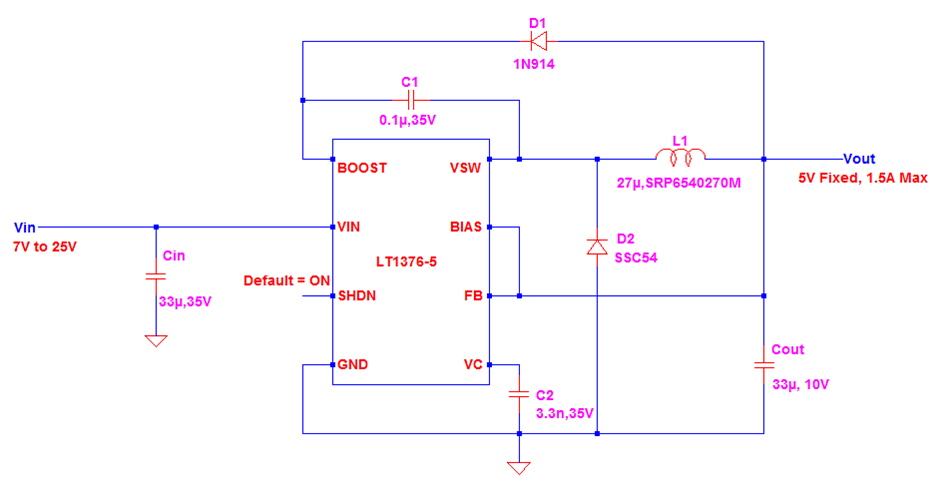# 7V to 25V Input with 5V and 1.5A Output Buck Converter Design Using LT1376-5 Controller

This design reference is for 7V to 25V input with 5V and 1.5A output buck converter using LT1376-5 controller. LT1376-5 is monolithic buck mode switching regulator which has a fixed frequency of 500 kHz. The switching element is internal on the controller thus lower component count. The internal switch can able to handle 1.5A current. The controller can be used with a wide range of input voltage from 6V to 25V. The output is fixed to 5V. There is also an adjustable variant that will be discussed in another article. This design example is concentrating to 7V to 25V input with 5V and 1.5A output buck converter.

This controller uses high switching frequency so smaller inductor and output capacitor size is possible. It uses a current mode control mechanism for better and fast transient response and has a good loop stability.

## 1. Design Specifications

Vin : 7V to 25V

Vout : 5V fixed

Iout_max : 1.5A

Output Ripple Voltage : 1% of the output voltage (peak to peak)

## 2. Circuit Schematic

Below is the circuit schematic of this design. For C1, the recommended value is 0.1uF. For C2, the recommended value is 3.3nF. We can use 33uF for Cin as a starting value and just trim in your actual design if the response is not perfect for your needs. You can use a diode with a current rating of at least 200mA for D1, for instance 1N914. We will select L1, D2 and Cout based from the computation results and also check the stress on the controller.## 3.Compute for Duty Cycle

We will compute the duty cycle for 7V and 25V inputs.## 4. Frequency and Period

LT1376-5 Controller has a fixed 500 kHz switching frequency. The corresponding period is 2µsec.## 5. Compute for the Minimum Inductance

To compute for the minimum inductance, the inductor ripple current must be declared. A rule of thumb for ripple current is 20-40% of the load current. If you want a very small ripple, you must set it as low as possible. Let us use here 20% only.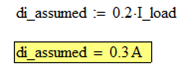Compute for the Inductance Value using 7V InputCompute for the Inductance Value using 25V InputThe computed minimum inductance at 25V input is to be used. If we will select the computed value using 7V input, the ripple at 25V input is high.

Let us use 27µH standard value inductor.

## 6. Recheck the Inductor Ripple Current using the Selected Inductor ValueWith the selected inductance value, the maximum inductor ripple current is happening at the maximum input voltage.

## 7. Compute for the Peak Current

We will compute for the peak current of the switch, inductor and the diode. Their peak currents are the same.## 8. Check the Operation Mode

For applications that are requiring smaller ripple current, CCM mode is more applicable. In CCM mode the inductor current is not touching zero in any switching cycle. In order to know of the operation is CCM, the minimum value of the inductor current must be above zero.The minimum inductor current is above zero using minimum and maximum input voltage. The operation is CCM indeed.

## 9. Check the Diode Current

`DC Value`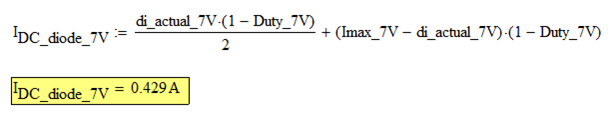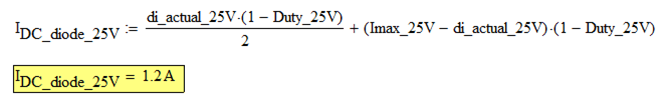`RMS Value`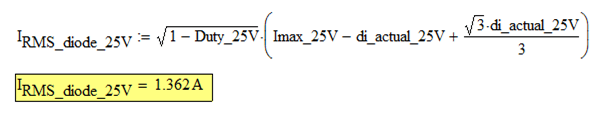## 10. Select a Diode

The maximum RMS current of the diode is only 1.35A. We will use here SSC54. This diode has a rated current of 5A and a voltage of 40V. Its maximum junction temperature is 150’C.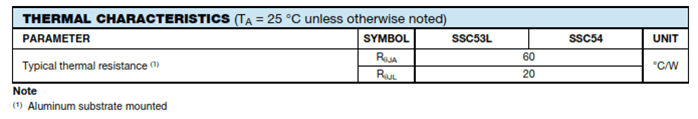The maximum power dissipated by the diode isConsidering the design is exposed to a 60’C maximum ambient temperature, the de-rated power capability of the diode isThe power stress of the diode at maximum operating ambient temperature is## 11. Check the Switch Current

`DC Value``RMS Value`From the datasheet, the minimum switch current limit is 1.5A for duty cycle less than 50% while 1.35A for duty cycle equal to 80%. Since the maximum computed duty cycle is 71%, we will use 1.35A as the reference.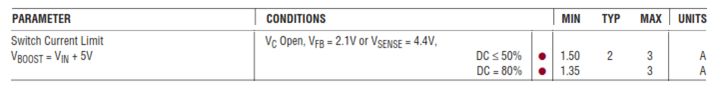The maximum computed switch current is only 1.272A, so the current limit will not hit and the converter can operate normally.

## 12. Check the Inductor Current

`RMS Current``DC Current`## 13. Select Inductor

The chosen inductance value in section 5 is 27µH. The computed peak current through the inductor is 1.648A as derived in section 7 while the maximum RMS current is 1.523A. From the table below, we can use 27µH with a RMS and saturation current rating of 2A which give us around 25% margin.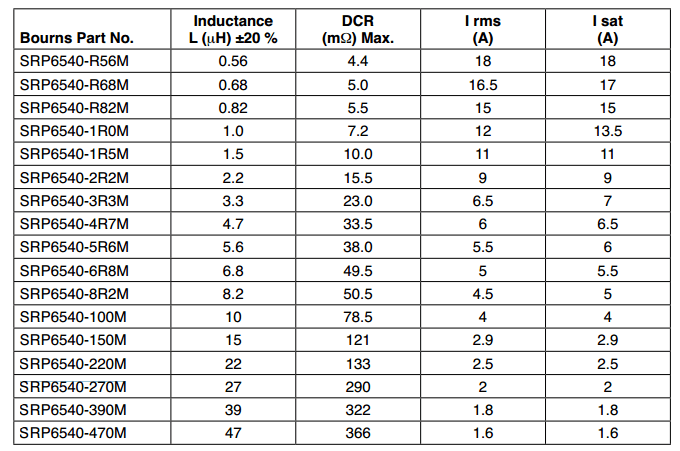## 14. Select the Minimum Output Capacitance Needed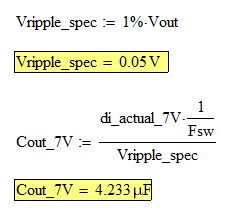We can use 33µF for more margin.

## 15. Final Schematic with Values1. Leonard says:

Nice one! Please is the input AC or DC?

2. Biswajit says:

How you are getting VQ1 voltage while calculating duty cycle ?

1. electronicsbeliever says:

During design, you can set VQ1 drop to zero for ideal assumptions. Anyways, its effect to the other parameters is insignificant. You can then verify during design verification testing.

3. Biswajit Singh says:

Hi sir

Could you please write a post on the current mode and voltage control mode. Its very confusing.

4. Biswajit says:

Dear sir
Could please post article on current mode control and voltage mode control ?

This site uses Akismet to reduce spam. Learn how your comment data is processed.